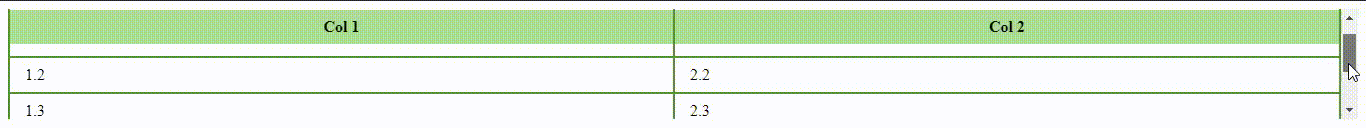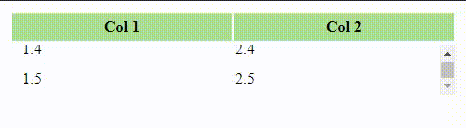Related Articles

# How to create a table with fixed header and scrollable body ?

• Last Updated : 25 Mar, 2021

1. By setting the position property to “sticky” and specifying “0” as a value of the top property for the <th> element.
2. By setting the display to “block” for both <thead> and <tbody> element so that we can apply the height and overflow properties to <tbody>.

Note:

• The position property states the type of positioning for an element.
• The display property states that how an element should be displayed.

Example: Below is the code which illustrates the formation of table with position property.

## HTML

 ```<``html``>`` ` `<``head``>``  ``<``style``>``    ``.fixTableHead {``      ``overflow-y: auto;``      ``height: 110px;``    ``}``    ``.fixTableHead thead th {``      ``position: sticky;``      ``top: 0;``    ``}``    ``table {``      ``border-collapse: collapse;        ``      ``width: 100%;``    ``}``    ``th,``    ``td {``      ``padding: 8px 15px;``      ``border: 2px solid #529432;``    ``}``    ``th {``      ``background: #ABDD93;``    ``}``  `````` ` `<``body``>``  ``<``div` `class``=``"fixTableHead"``>``    ``<``table``>``      ``<``thead``>``        ``<``tr``>``          ``<``th``>Col 1``          ``<``th``>Col 2``        ````      ```` ` `      ``<``tbody``>``        ``<``tr``>``          ``<``td``>1.1``          ``<``td``>2.1``        ````        ``<``tr``>``          ``<``td``>1.2``          ``<``td``>2.2``        ````        ``<``tr``>``          ``<``td``>1.3``          ``<``td``>2.3``        ````        ``<``tr``>``          ``<``td``>1.4``          ``<``td``>2.4``        ````        ``<``tr``>``          ``<``td``>1.5``          ``<``td``>2.5``        ````      ````       ` `    ````  `````` ` ``

Output:Example 2: Below is the code which illustrates the formation of table with display property.

## HTML

 ```<``html``>`` ` `<``head``>``  ``<``style``>``    ``.tableFixHead {``      ``width: 500px;``      ``table-layout: fixed;``      ``border-collapse: collapse;``    ``}``      ``.tableFixHead tbody {``      ``display: block;``      ``width: 100%;``      ``overflow: auto;``      ``height: 50px;``    ``}``    ``.tableFixHead thead tr {``      ``display: block;``    ``}``    ``.tableFixHead th,``    ``.tableFixHead  td {``      ``padding: 5px 10px;``      ``width: 200px;``    ``}``    ``th {``      ``background: #ABDD93;``    ``}``  `````` ` `<``body``>``  ``<``div` `class``=``"tableFixHead"``>``    ``<``table``>``      ``<``thead``>``        ``<``tr``>``          ``<``th``>Col 1``          ``<``th``>Col 2``        ````      ````      ``<``tbody``>``        ``<``tr``>``          ``<``td``>1.1``          ``<``td``>2.1``        ````        ``<``tr``>``          ``<``td``>1.2``          ``<``td``>2.2``        ````        ``<``tr``>``          ``<``td``>1.3``          ``<``td``>2.3``        ````        ``<``tr``>``          ``<``td``>1.4``          ``<``td``>2.4``        ````        ``<``tr``>``          ``<``td``>1.5``          ``<``td``>2.5``        ````      ````    ````  `````` ` ``

Output:Attention reader! Don’t stop learning now. Get hold of all the important HTML concepts with the Web Design for Beginners | HTML  course.

My Personal Notes arrow_drop_up